## Меню

Главная
Финансы деньги и налоги
Издательское дело и полиграфия
Новейшая история политология
Топики по английскому языку
Языкознание филология
Химия
Экология и охрана природы
Естествознание
Искусство и культура
Культурология
Хозяйственное право
Иностранные языки и языкознание
История и исторические личности
Коммуникации связь цифровые приборы и радиоэлектроника
Товароведение
Транспорт
Экология охрана труда
Банковское и биржевое дело
Коммуникации и связь
Конституционное право
Этика

##### Научно-образовательный портал W-10.RU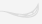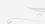Главная

# Is the nature of quantum chaos classical?

## Is the nature of quantum chaos classical?

K.N. Yugay, S.D. Tvorogov, Omsk State University, General Physics Department,  pr.Mira,55-A 644077 Omsk, RUSSIA  Institute of Atmosphere Optics of Russian Academy of Sciences

Recently discussions about what is a quantum chaos do not abate [1-16]. Some authors call in question the very fact of an existence of the quantum chaos in nature . Mainly reason to this doubt is what the quantum mechanics equations of motion for the wave function or density matrix are linear whereas the dynamical chaos can arise only into nonlinear systems. In this sence the dynamical chaos in quantum systems, i.e. the quantum chaos, cannot exist. However a number of experimental facts allow us to state with confidence that the quantum chaos exists. Evidently this contradiction is connected with what our traditional description of nature is not quite adequate to it.

Reflecting on this problem one cannot but pay attention to the following:

i) two regin exist - the pure quantum one (QR) and the pure classical one (CR), where descriptions are essentially differed. The way in which the quantum and classical descriptions are not only two differen levels of those, but it seems to be more something greater; the problem of quantum chaos indicates to it. Since experimental manifestations of quantum chaos exist therefore one cannot ignore the question on the nature of quantum chaos and the description of it.

ii) It undoubtedly that the intermediate quantum-classical region (QCR) exists between the QR and the CR, which must be possessed of characteristics both the QR and the CR. Since the term "quasiclassics" is connected traditionally with corresponding approximate method in the quatum mechanics we shall call this region as quantum-classical one further. It is evident that the QCR is the region of high excited states of quantum systems.

Below shall show that quantum and classical problems are not autonomous into the QCR but they are coupled with each other, so that a solution of a quantum problem contains a solution of a corresponding classical problem, but not vice versa.

A possible dynamical chaos of a nonlinear classical problem has an effect on the quantum problem so that one can say quantum chaos arises from depths of the nonlinear classical mechanics and it is completely described in terms of nonlinear dynamics, for example, instability, bifurcation, strange attractor and so on. We shall show also that the connection between the quantum and classical problems is reflected on a phase of a wave function which having a quite classical meaning is subjected to its classical equation of motion and in the case of its nonlinearity into the system the dynamical chaos is excited.

One of a splendid example of a role of the wave function phase is a description of dynamical chaos in a long Josephson junction [17-24]. Here the wave function phase (the difference phases on a junction) of a superconducting condensate is subjected to the nonlinear dynamical sine-Gordon equation. The dynamical chaos arising in a long Josephson junction and describing by the sine-Gordon equation is a quantum chaos essentially since the question is about a phenomenon having exceptionally the quantum character. However the quantum chaos is described here precisely by the classical nonlinear equation.

Below we shall try to show that the description of the quantum chaos in the more general case may be carry out just as in a long Josephson junction in terms of nonlinear classical dynamics equations of motion to wich the wave function phase of a quantum is subjected. In addition the quantum system must be into the QCR, i.e. into high excited states.

Let us assume that the Hamiltonian of a system have the form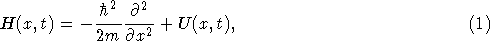where the operator of the potential energy U(x,t) is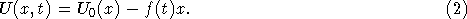(We examine here an one-dimensional system for the simplicity). Here U0(x) is the nonperturbation potential energy, and f(t) is the time-dependent external force.

We shall found the solution of the Schrödinger equation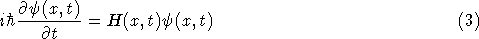in the form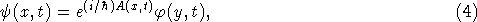where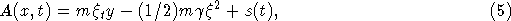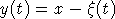,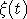is the solution of the classical equation of motion,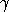is the certain constant, s(t) is the time-dependent function, the sense of that will be clear later on. We notice that the function A(x,t) is real. (A representation of the phase A(x,t) in the form (5) at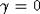was introduced first by Husimi ).

Substituting (4) into Eq.(1) and taking into account (5), we get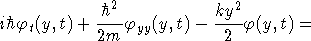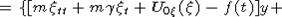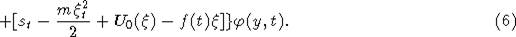Here subscripts t, y anddenote the partial derivatives with respect to time t and coordinates y,, respectively.

On the right of Eq.(6) the expressions of both square brackets are equal to zero because of following relations:

i) of the classical equation of motion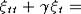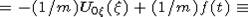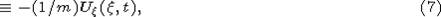where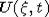is the same potential, that is into (3), and

ii) of the expression for the classical Lagrang function L(t)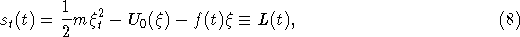so that the function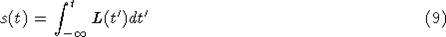makes a sense of an action integral.

Into Eq.(6)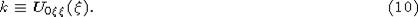By deduction of Eq.(6) we made use of an potential energy expansion in the form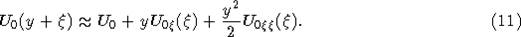It is obvious that the expansion (11) is correct in the case when a classical trajectory is close to a quantum one.

Thus we get the equation for the function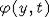in the form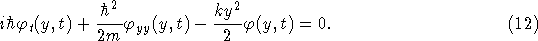We pay attention here to three originating moments: 1) Equation (12) is the Schrödinger equation again, but without an external force. 2) We have the system of two equations of motion: quantum Eq.(12) and classical Eq.(7). In a general case these equations make up the system of bound equations, because the coefficient k can be a function of classical trajectory,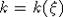. As we show below a connection between Eqs. (12) and (7) arises in the case, if classical Eq. (7) is nonlinear. 3) Classical Eq.(7) contains some dissipative term, and somakes sense of a dissipative coefficient. The arising of dissipation just into the classical equation is looked quite naturally - a dissipation has the classical character.

Let us assume that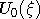is the potential energy of a linear harmonic oscillator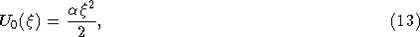whereis the certain constant. Then we have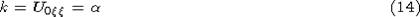and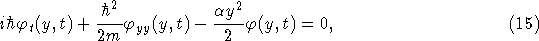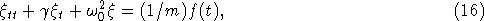where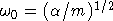is the natural frequency of the harmonic oscillator. Equations (15) and (16) represent the corresponding equations of the quantum and classical linear harmonic oscillators. We see that Eqs.(15) and (16) are autonomous with respect to each other. Thus in the case if the classical limit (16) of the corresponding quantum problem (15) is linear then the solution of the classical and quantum one are not connected with each other.

Let us assume now that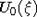have a form of the potential energy of the Duffing oscillator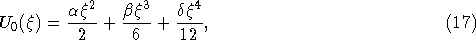where,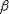andare some constants. For the potential energy (17) k takes the form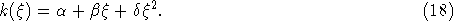Then we have the following equations of motion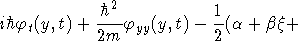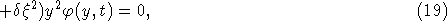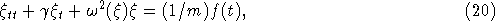where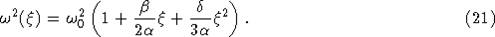Equation (20) represents the equation of motion for a nonlinear oscillator. It is seen, that quantum (19) and classical (20) equations of motion are coupled with each other.

We return to the discussion of expansion (11). It is seemed obvious, that the classical and quantum trajektories coexist and close to each other only into the QCR. Into the pure quantum region QR and into the pure classical one CR these trajectories cannot coexist: because into the CR a de Broglie wave packet fails quickli in consequence of dispersion; into the QR the classical trajectory dissappears in consequence of uncertainty relations. Thus expansion (11) is correct into the quantum-classical region QCR only, or in other words into the quasiclassical region. The QCR is became essential just in cases when a classical problem proves to be nonlinear.

The transition of a particle from the low states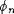(from the QR) into high excited states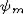(into the QCR) is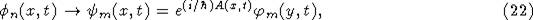where A(x,t) is defined with the expression (5). It is easily seen that the probability of this transition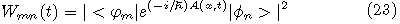will be depend on the solution of the classical equation of motion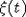.

Since the classical problem (19) is nonlinear, then into its, as it is known  dynamical chaos can be arisen. This chaos will lead to nonregularities in the wave function phase A(x,t) and also in the function, that in turn will lead to nonregularities of the probabilities of the transition in high excited states, and also from high excited states into states of the continuous spectrum. In this way it can be said that the quantum chaos is the dynamical chaos in the nonlinear classical problem, defining quantum solutions, from the point of view of the stated here theory.

These investigations are supported by the Russian Fund of Fundamental Researches (project No. 96-02-19321).

Список литературы

Zaslavsky G.M., Chirikov B.V. Stochastic Instability of Nonlinear Oscillations // Usp. Fiz. Nauk. 1971. V.105. N.1. P.3-29.

Chirikov B.V., Izrailev F.M., Shepelaynsky D.L. Dynamical Stochasticity in Classical and Quantum Mechanics // Sov. Sci. Rev., Sect.C. 1981. V.2. P.209-223.

Toda M., Ikeda K. Quantum version of resonance overlap //J.Phys.A: Math. and Gen. 1987. V.20. N.12. P.3833-3847.

Nakamura K. Quantum chaos. Fundamental problems and application to material science // Progr. Theor. Phys. 1989. Suppl. N.98. P.383-399.

FloresJ.C. Kicked quantum rotator with dynamic disorder. A diffusive behaviour in momentuum space // Phys. Rev. A. 1991. V.44. N.6. P.3492-3495.

Gasati G., Guarneri I., Izrailev F., Scharf R. Scaling behaviour of localization in quatum chaos // Phys.Rev.Lett. 1990. V.64. P.5-8.

Chirikov B.V. Time-dependent Quantum Systems.- In 'Chaos and Quantum Physics', eds. M.-J. Giannoni, A. Voros, J. Zinn-Justin. Proc. Les. Houches Summer School. Elsevier, 1991. P.445.

Chirikov B.V. Chaotic Quantum Systems. Prepr. 91-83. Novosibirsk, 1991.

Elutin P.V. A Problem of Quantum Chaos // Usp. Fiz. Nauk. 1988. V.155. N.3. P.397-442.

Berman G.P., Kolovsky A.R. Quantum Chaos in interactions of multilevel quantum systems with a coherent radiation field // Usp. Fiz. Nauk. 1992. V.162. N.4. P.95-142.

Heiss W.D., Kotze A.A. Quantum Chaos and analytic structure of the spectrum // Phys. Rev. A. 1991. V.44. N.4. P.2401-2409.

Prosen T., Robnic M. Energy level statistics and localization in sparced banded random matrix ensemble // J. Phys. A. 1993. V.26. N.5. P.1105-1114.

Berry M.V., Keating J.P. A rule for quantizing chaos? // J. Phys. A. 1990. V.23. N.21. P.4839-4849.

Saito N. On the origin and nature of quantum chaos // Progr. Theor. Phys. 1989. Suppl. N.98. P.376-382.

Ben-Tal N., Moiseyev N., Korsch H.J. Quantum versus classical dynamics in a periodically driven anharmonic oscillator // Phys. Rev. A. V.46. N.3. P.1669-1672.

Yugay K.N. On quantum problems nonlinear in classical limit //Izv. Vuzov. Physica. 1992. N.7. P.104-107.

Cirillo M., Pedersen N.F. On bifurcations and transition to chaos in Josephson junction // Phys. Lett. A. 1982. V.90. N.3. P.150-152.

Dalsgaard J.H., Larsen A., Mygind J. Chaos in self-pumped resonator coupled Josephson junctions // Physica B. 1990. V.165-166. N.2. P.1661-1662.

Yeh W.J., Symko O.G., Zheng D.J. Chaos in long Josephson junctions without external rf driving force // Phys. Rev. B, 1990. V.42. N.7. P.4080-4087.

Yao X., Wu J.Z., Ting C.S. Onset of chaos in Josephson junctions with intermediate dumping // Phys. Rev. B. 1990. V.42. N.1A. P.244-250.

Rajasekar S., LakshmananM. Multiple attractors and their basins of attraction of a long Josephson junction // Phys. Lett. A. 1990. V.147. N.5-6. P.264-268.

Gronbech-Jensen N., Lomdahl P.S., Samuelsen M.R. Bifurcation and chaos in a dc-driven lomg annular Josephson junction // Phys. Rev. B. 1991. V.43. N.16. P.12799-12803.

Guerrero L.S., Ostavio M. Quasiperiodic route to soft turbulence in long Josephson junctions // Physica B. 1990. V.165-166. N.2. P.1657-1658.

Yugay K.N., Blinov N.V., Shirokov I.V. Effect of memory and dynamical chaos in long Josephson junctions // Phys. Rev. B. 1995. V.51. N.18. P.12737-12741.

Husimi K.// Progr. Theor. Phys. 1953. V.9. P.381.

Lichtenberg A.J., Lieberman M.A. Regular and Stochastic Motion. Springer-Verlag, New-York, 1983.

Для подготовки данной работы были использованы материалы с сайта http://www.omsu.omskreg.ru/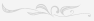## Поиск

Расширенный поиск